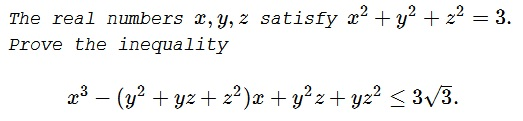# 6th European Mathematical Cup (2017), Junior Problem 4

### Problem### Solution

Observe that, according to Viète's formulas, the polynomial

\begin{align} f(x)&= x^3 - (y^2 + yz + z^2)x + y^2z+yz^2\\ &=x^3 - [yz-y(y+z)-z(y+z)]x + yz(y + z) \end{align}

has three real roots: $y,z,-(x+y).$ It follows that $f(x)=(x-y)(x-z)(x+y+z),$ making the required inequality equivalent to

$\displaystyle (x-y)^2(x-z)^2(x+y+z)^2 \le (x^2+y^2+z^2)^3.$

By the AM-GM inequality,

\displaystyle\begin{align}(x-y)^2(x-z)^2(x+y+z)^2&\le \left(\frac{(x-y)^2+(x-z)^2+(x+y+z)^2}{3}\right)^3\\ &=\left(\frac{3x^2+2y^2+2z^2+2yz}{3}\right)^3, \end{align}

with equality iff $(x-y)^2=(x-z)^2=(x+y+z)^2.$ Thus suffice it to prove that

$3x^2+2y^2+2z^2+2yz\le3x^2+3y^2+3z^2,$

which is just $(y-z)^2\ge 0,$ with equality for $y=z.$ All the equality conditions hold for $y=z=0$ and $x=\sqrt{3},$ or $y+2x=0,$ i.e., $\displaystyle x=-\frac{1}{\sqrt{3}},$ $\displaystyle y=z=\frac{2}{\sqrt{3}}.$

### Acknowledgment

This is Problem 4 (by Miroslav Marinov) from the 2017 European Mathematical Cup (Junior category). I am grateful to Leo Giugiuc for bringing this competition and the problem to my attention and for supplying the beautiful solution above.